0
690

# Directions Questions For LIC AAO PDF

Download Important Direction sense test questions for LIC AAO exam. Top 20 Reasoning directions questions with answers based on previous year asked questions.

Question 1: How many such pairs of letters are there in the word ‘PLATFORMS’ each of which as many letters between them in the word (in both forward and backward directions), as they have between them in the English alphabetical series?

a) Three

b) None

c) One

d) Two

e) More than three

Instructions

Study the following information carefully and answer the questions given below :

Eight persons — A, B, C, D, P, Q, R and S — are sitting around a circular table with equal distances between each other (but not necessarily in the same order). Some of them face outside and some others face the centre.
NOTE : Facing the same direction means if one faces the centre then the other also faces the centre and viceversa. Facing the opposite directions means if one faces the centre then the other faces outside and viceversa. Immediate neighbours face the same direction means if one neighbour faces the centre then the other neighbour also faces the centre and viceversa. Immediate neighbours face the opposite directions means if one neighbour faces the centre then the other neighbour faces outside and viceversa. P sits to the immediate right of C. Only three persons sit between P and A. R sits third to the left of A. Neither A nor C is an immediate neighbour of B. D sits to the immediate left of B. Q sits second to the left of D. The immediate neighbours of A face the same direction. The immediate neighbours of B face opposite directions. S sits second to the right of P. The immediate neighbours of C face the same direction. S faces outside

Question 2: Who sits exactly between B and the one who sits to the immediate left of R, when
counted from the right of B?

a) D

b) A

c) P

d) R

e) Q

Question 3: How many persons in the given arrangement face the centre?

a) More than four

b) One

c) Three

d) Two

e) Four

Question 4: Who sits third to the right of Q?

a) B

b) P

c) A

d) Other than those given as options

e) R

Question 5: What is the position of D with respect to S?

a) Second to the right

b) Third to the right

c) Third to the left

d) Immediate right

e) Fourth to the left

Question 6: Four of the following five are alike in a certain way based on the given sitting arrangement and so form a group. Which is the one that does not belong to that group?

a) P

b) Q

c) D

d) B

e) A

Question 7: How many such pairs of letters are there in the word OVER SEE’ each of which has as many letters between them in the word (in both forward and backward directions) as they have between them in the English alphabetical series ?

a) Three

b) One

c) Two

d) None

e) More than three

Question 8: How many such pairs of letters are there in the word ‘CHARGED’, each of which has as
many letters between them in word (in both forward and backward directions) as in the
English alphabetical series ?

a) None

b) One

c) Two

d) Three

e) More than three

Question 9: A travel towards East. B travels towards North. C and D travel in opposite directions. D travels towards right of A. Which of the following is definitely true?

a) B and C travel in opposite directions

b) C travels towards West

c) D travels towards North

d) B and C travel in the same direction

e) None of these

InstructionsDirections : Study the following information carefully and answer the given questions.

Question 10: A school bus driver starts from the school, drives 2 km towards North, takes a left turn and drives for 5 km. He then takes a left turn and drives for 8 km before taking a left turn again and driving for further 5 km. The driver finally takes a left turn and drives 1 km before stopping. How far and towards which direction should the driver drive to reach the school again?

a) 3km towards North

b) 7 km towards East

c) 6 km towards South

d) 6 km towards West

e) 5 km towards North

Question 11: If all the directions are rotated by 45 degrees in the clockwise direction, followed by 135 degrees in the anti-clockwise direction, followed by 270 degrees in the clockwise direction, which original direction does the new southeast point to?

a) northeast

b) east

c) north

d) northwest

e) southwest

Question 12: If South-West becomes East, then what will South-East become ?

a) South

b) North -East

c) West

d) North

e) Can’t be determined

Question 13: A man after walking 8km turned to his right and walked for 5 more km. Then he turned right and walked 2 km. After that he walked 8 km to the left and was facing North East. In which direction did he start initially ?

a) South-East

b) North

c) North-West

d) South-West

e) None of the above

Question 14: If South-West becomes East, then what will South-East become ?

a) South

b) North -East

c) West

d) North

Question 15: A man after walking 8km turned to his right and walked for 5 more km. Then he turned right and walked 2 km. After that he walked 8 km to the left and was facing North East. In which direction did he start initially ?

a) South-East

b) North

c) North-West

d) South-West

Question 16: Ram is standing to the West of Shyam. Shyam is standing to the North of Vaibhav. Vaibhav is standing to the SouthEast of Kuru. In which direction does Kuru have to look to find Ram?

a) North East

b) North West

c) South East

d) South West

e) Can’t be determined

Instructions

Answer the following questions based on this information.

Mitesh starts from his home and walks 2 km in North direction. He then takes a right turn and walk another 2 km. Then he takes a left and walks 1 km. Finally he takes a left again and walks another 1 km and stops at that point.

Question 17: What is the direction he is finally facing?

a) North

b) West

c) East

d) South

e) Cannot be determined

Question 18: What is the shortest distance between his final position and his house?

a) $3\sqrt{2}$ km

b) $2\sqrt{3}$ km

c) $2\sqrt{5}$ km

d) $\sqrt{10}$ km

e) None of these

Instructions

The next two questions are based on the following information.
Rahul starts from his home and walks 4 km in east direction. He then takes a left turn and walks 5 km. He then takes a left again and walks 28 km. Finally, he takes a right turn and walks 2 km and stops.

Question 19: What is the minimum distance between his initial position and his final position?

a) $\sqrt{527}$ km

b) $25$ km

c) $\sqrt{833}+4$ km

d) $21$ km

e) None of these

Question 20: What direction is Rahul finally facing?

a) South

b) East

c) West

d) North

e) South-east

TECHNCIAL SKILLS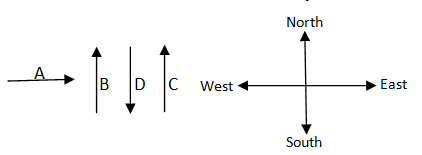The driver drove 5 km west, 5 km east, 8 km south and 2+1 = 3 km north. So, he has to travel a distance of 8 – 3 = 5 km north to reach his starting point.

Consider the southeast direction. After the 45 degree clockwise rotation, it points to south. After the 135 degree anti-clockwise rotation, it points to northeast. After the 270 degree clockwise rotation, it points to northwest direction.

iven, South West is East. So we draw a new direction chart in which South-West is marked as East. Next, we mark West which is opposite East and then North which is 90$^0$ anti-clockwise of East. After that South is marked which is opposite to North. Once we know the main 4 directions, the 4 sub directions are marked as normal.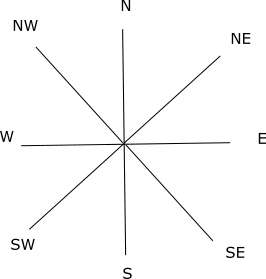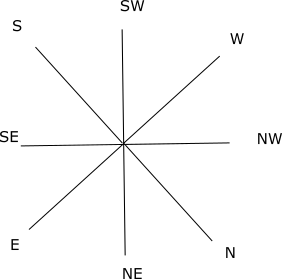From the figure, we can see that South-East will become North.

Since the initial direction of the man is not given, assume the initial direction as East. By following the directions given in the question the man will be facing South at the end. The figure is as follows: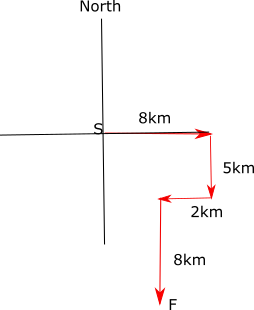But the final direction of the man is given as North East. Thus, the direction we have taken as South is the actual North-East. From the figure given below we can see that the initial direction South which we have considered is actually the North-West direction.iven, South West is East. So we draw a new direction chart in which South-West is marked as East. Next, we mark West which is opposite East and then North which is 90$^0$ anti-clockwise of East. After that South is marked which is opposite to North. Once we know the main 4 directions, the 4 sub directions are marked as normal.From the figure, we can see that South-East will become North.

Since the initial direction of the man is not given, assume the initial direction as East. By following the directions given in the question the man will be facing South at the end. The figure is as follows:But the final direction of the man is given as North East. Thus, the direction we have taken as South is the actual North-East. From the figure given below we can see that the initial direction South which we have considered is actually the North-West direction.Just with the given information and without the exact distances between them, we can’t identify the directions between the persons because depending on the distances, the actual directions vary.

Consider the following figure.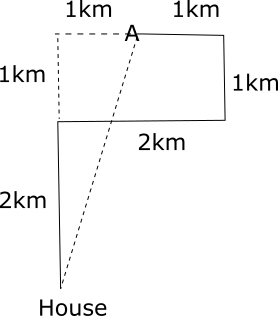Since he started in the north direction, his final direction should be West.

Consider the following figure.The shortest path is along the dotted line.
Horizantal distance = 2-1 = 1 km
Vertical distance = 2+1 = 3 km
Using Pythagoras theorem we can find out the length of the hypotenuse which is the shortest distance.
This shortest distance will be $\sqrt{3^2 + 1^2}=\sqrt{10}$

Consider the following figure-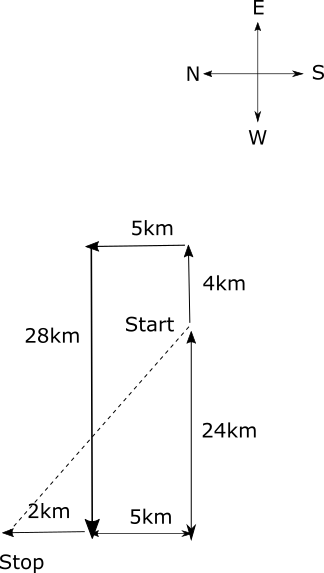The minimum distance is along the dotted lines i.e. $\sqrt{24^2+7^2}=25$ km